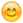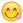# Changing order of integration

## Homework Statement

Switch the order of integration:
∫∫f(x,y) dydx, with 0≤x≤π/2, 0≤y≤sin(x)

## The Attempt at a Solution

∫∫f(x,y) dxdy, with sin(x)≤x≤π/2, 0≤y≤sin(x)

The upper bound of x is π/2, while the lower bound is sin(x). It's just the first 1/4 of the area of a sine wave. The upper bound of y is sin(x) and lower bound is 0.

I was just wondering if someone could confirm. I've been having a bit of trouble with integrals and assigning regions. Thank you :)

Mark44
Mentor

## Homework Statement

Switch the order of integration:
∫∫f(x,y) dydx, with 0≤x≤π/2, 0≤y≤sin(x)

## The Attempt at a Solution

∫∫f(x,y) dxdy, with sin(x)≤x≤π/2, 0≤y≤sin(x)

The upper bound of x is π/2, while the lower bound is sin(x).
No. In the first integral above, you are looking at vertical strips that go from y = 0 to y = sin(x), and summing them as x ranges from x = 0 to x = pi/2.
says said:
It's just the first 1/4 of the area of a sine wave. The upper bound of y is sin(x) and lower bound is 0.
No. Switching the order of integration means that you will now be using horizontal strips, and summing them from bottom to top.
What is the leftmost x-value on a given horizontal strip? (The rightmost x-value is pi/2.)
You need to be thinking about inverse functions.
says said:
I was just wondering if someone could confirm. I've been having a bit of trouble with integrals and assigning regions. Thank you :)

The rightmost value is pi/2, so the leftmost value would be sin(x), no?

Or should I be saying the leftmost value is 0?

I'm saying sin(x) because when we sub in sin(0) we get 0.

LCKurtz
Homework Helper
Gold Member
Think of a little dydx square in the middle of your area. Now move it left and right. Your inner integral should look like ##\iint_{x_{left}}^{x_{right}}f(x,y)~dxdy##. When you move that dydx square to the left it hits the sine curve. What is the x value there in terms of y? The value sin(x) you suggested in post #3 is the y value, not the x value.

Mark44
Mentor
Or should I be saying the leftmost value is 0?

I'm saying sin(x) because when we sub in sin(0) we get 0.
Have you drawn a sketch of the region over which integration is being performed? If not, you really should.

Also, instead of saying "leftmost value," it's better to specify leftmost x value, as well as lower y value and upper y value.

I drew a picture. Always the first thing I do.

The leftmost x value, judging by my picture, = 0, while the rightmost value = pi/2
The upper y value = sin(x) while the lower y value = 0

0≤x≤pi/2
0≤y≤sin(x)

#### Attachments

•photo (2).JPG
25.5 KB · Views: 423
Mark44
Mentor
I drew a picture. Always the first thing I do.

The leftmost x value, judging by my picture, = 0, while the rightmost value = pi/2
For the shaded region, is the leftmost x value 0 for each point on the left edge of the region?
says said:
The upper y value = sin(x) while the lower y value = 0

0≤x≤pi/2
0≤y≤sin(x)
Look at what LCKurtz wrote a few posts back.

opps, please refer to post #7 :)

LCKurtz
Homework Helper
Gold Member
I drew a picture. Always the first thing I do.

The leftmost x value, judging by my picture, = 0, while the rightmost value = pi/2
The upper y value = sin(x) while the lower y value = 0

0≤x≤pi/2
0≤y≤sin(x)

Which makes me think you haven't read my post #5.

Oh, so I want the leftmost value for the whole region. I was just writing down 0 because 0 is the leftmost point!

The leftmost x=sin(x) and the rightmost pi/2
the upper y = sin(x) and lower = 0

How is this different from my original answer though?

sin(x)≤x≤pi/2
0≤y≤sin(x)

Mark44
Mentor
says said:
Switch the order of integration:
∫∫f(x,y) dydx, with 0≤x≤π/2, 0≤y≤sin(x)
The two inequalities should really be 0 ≤ y ≤ sin(x), 0 ≤ x ≤ π/2
In the original integral, you are using vertical strips, each of which ranges from y = 0 to y = sin(x). The outer integral sums all of these vertical strip areas, as x ranges between 0 and π/2.

Switching the order of integration means you need a different description of the region. In this integral, the inner integral uses horizontal strips, each of which ranges from x = ##x_{left}## to x = ##x_{right}##. The horizontal strip areas are then summed as y ranges from 0 to 1.

You need to figure out what ##x_{left}## and ##x_{right}## are. I'm pretty much repeating what I said in post #2.

Note: I've been a little sloppy by saying "area" above. Since the integrand contains a function, you aren't actually computing the area.

Mark44
Mentor
The leftmost x=sin(x)
x = sin(x) makes no sense here.

For the equation y = sin(x), if you know an x value, it's easy to get the y value. However, what if you know the y value? How do you get the x value on the same graph?
says said:
and the rightmost pi/2
the upper y = sin(x) and lower = 0

How is this different from my original answer though?

sin(x)≤x≤pi/2
0≤y≤sin(x)
It's not different -- that's the problem, since your first answer was wrong.

0 ≤ y ≤ sin(x), 0 ≤ x ≤ π/2

if x=0 then sin(0) = 0, so how come sin(x)≤x≤pi/2 can't be a valid region also?
I don't understand what you mean by saying x = sin(x) makes no sense?

Ignore that last question! I understand now. Thanks :)

LCKurtz
Homework Helper
Gold Member

∫∫f(x,y) dxdy, with 0 ≤ x ≤ π/2, 0 ≤ y ≤ sin(x) being the lower and upper bounds of integration.

Mark44
Mentor
∫∫f(x,y) dxdy, with 0 ≤ x ≤ π/2, 0 ≤ y ≤ sin(x) being the lower and upper bounds of integration.
No. All you really have done is switch dx and dy in the integral, and swap the order of the inequalities. Go back and read the replies in posts 2, 5, 10, 12, 15, and 16.

It's just that one lower bound x value I'm confused with.

arcsin?

LCKurtz
Homework Helper
Gold Member
He's all yours Mark44. I have something I have to do the next few hours.

Mark44
Mentor
I said in an earlier post that the leftmost x was sin(x), to which you said that made no sense, which i understand. You also mentioned inverse functions.

∫∫f(x,y) dxdy, with arcsin ≤ x ≤ π/2, 0 ≤ y ≤ sin(x)
arcsin all by itself isn't a number, but you're on the right track in the first set of inequalities. For the second set, the horizontal strips range from y = 0 to y = ?

•says
NascentOxygen
Staff Emeritus
With all the digital technology on offer, Maths can now more than ever be explored as a discovery science.You could use wolframalpha to confirm your answer. For example, I've nominated f(x,y) of my choosing and evaluated it between the same boundaries as in your exercise.
http://m.wolframalpha.com/input/?i=integrate+x^2+xy+from+y=0+to+sin(x)+from+x=0+to+Pi/2&x=0&y=0

Then, after working out what you think is the way to perform it with order reversed, give it to wolframalpha to evaluate and see whether this answer agrees with the first.

I tried it, and incorporating arcsin it worked.∫∫f(x,y) dxdy, with arcsin y ≤ x ≤ π/2, 0 ≤ y ≤ 1

Mark44
Mentor
∫∫f(x,y) dxdy, with arcsin y ≤ x ≤ π/2, 0 ≤ y ≤ 1
Yes!

•says